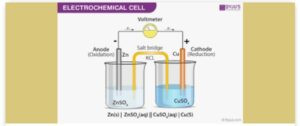You dont have javascript enabled! Please enable it!

# Electrochemical Cell Calculation And Types

## Electrochemical Cell Calculation And Types

Electrochemical Cell Calculation And Types.

ELECTROCHEMICAL CELL
CALCULATIONS OF E.M.F OF A CELL:
The E.M.F of a cell is calculated using the formula below
E0 Cell [EMF] = E0 (right)- E0(left).
NB. The half cell with the most negative or less positive electrode potential (E.P.) should always be shown as the left-hand half cell. Examples:1. Calculate the EMF of the cell below
Cu2+/ Cu; E0 = +0.34V
Zn2+/ Zn; E0 = – 0.76V.
EMF (E0 cell) = E0right – E0left
= E0Cu2+ / Cu – E0 Zn2+/Zn
= 0.34 – (-0.76)
=+ 1.10V
2. What is the EMF of a cell with the following half cell.
Ag+/ Ag; E0 = +0.80V
Cu2+/Cu; = + 0.34V
EMF = E0RIGHT – E0LEFT
E0Ag+/Ag – E0Cu2+ Cu
= 0.80 – (+0.34)
= + 0.46V.

### Types of Electrochemical Cells

There are two types of electrochemical cells namely:
1. Primary cell and
2. Secondary cell.
Primary cells are the type of cell in which electric current is produced by using up completely their chemical component. It can be recharged and therefore thrown away and replaced, with a new one. It does not supply a steady current e.g. Daniel cell, le clancle cell, torch battery, radio clock battery, etc.
• Le clanche cell: le clanche cell has a wide range of uses in small electrical appliances, such as torch battery, radio battery, clock battery. At the anode, zinc atoms loss two electrons to dissolve in the electrolyte as positively charged ions.
Zn(s) → Zn2+ + 2e-

At the cathode, the ammonium ions accept electron from the graphite rod and become reduced to ammonia and hydrogen:
2NH4+(aq) + 2e- → 2NH3(g) + H2(g)
The cell diagram is represented by : Zn(s) / zn2+, NH4+(aq) → 2NH3(g) + H2.
The hydrogen gas is removed by manganese (iv) oxide which acts as a depolarizer. Hydrogen gas causes polarization of the cell. The le clanche cell is of two types namely: dry le clanche cell and wet l eclanche cell.
The dry cell is called dry because the electrolyte is a paste of ammonium chloride and starch while the wet cell contains a solution of ammonium chloride.
Secondary cell: the secondary cell is the cell that can be recharged when it runs down. This is done by passing a direct current through it. Secondary cells are lead-acid accumulator (car battery).
Difference between Primary cell and Secondary cell
Primary cell Secondary cell
Primary cell cannot be recharged Secondary cell can be recharged
It is irreversible It cannot be reversible
Difference between the electrolytic cell and electrochemical cells
Electrochemical cell.

• Chemical reaction produces electricity Current is required for the reaction to occur.
• Cathode is the positive electrode Cathode is the negative electrode
• Anode is a negative electrode Anode is the positive electrode
• Electrode are in separate compartment Electrodes are in the same compartment

RECOMMENDED FOR YOU

Acid Base And Salt – Bases And Alkalies

Mineral Resources In Nigeria And Their Uses